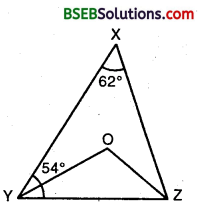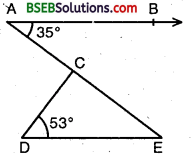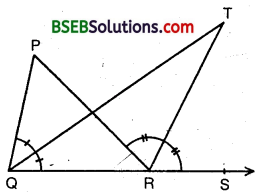# HSSlive: Plus One & Plus Two Notes & Solutions for Kerala State Board

## BSEB Class 9 Maths Chapter 6 Lines and Angles Ex 6.3 Textbook Solutions PDF: Download Bihar Board STD 9th Maths Chapter 6 Lines and Angles Ex 6.3 Book AnswersBSEB Class 9 Maths Chapter 6 Lines and Angles Ex 6.3 Textbook Solutions PDF: Download Bihar Board STD 9th Maths Chapter 6 Lines and Angles Ex 6.3 Book Answers

BSEB Class 9th Maths Chapter 6 Lines and Angles Ex 6.3 Textbooks Solutions and answers for students are now available in pdf format. Bihar Board Class 9th Maths Chapter 6 Lines and Angles Ex 6.3 Book answers and solutions are one of the most important study materials for any student. The Bihar Board Class 9th Maths Chapter 6 Lines and Angles Ex 6.3 books are published by the Bihar Board Publishers. These Bihar Board Class 9th Maths Chapter 6 Lines and Angles Ex 6.3 textbooks are prepared by a group of expert faculty members. Students can download these BSEB STD 9th Maths Chapter 6 Lines and Angles Ex 6.3 book solutions pdf online from this page.

## Bihar Board Class 9th Maths Chapter 6 Lines and Angles Ex 6.3 Books Solutions

 Board BSEB Materials Textbook Solutions/Guide Format DOC/PDF Class 9th Subject Maths Chapter 6 Lines and Angles Ex 6.3 Chapters All Provider Hsslive

## How to download Bihar Board Class 9th Maths Chapter 6 Lines and Angles Ex 6.3 Textbook Solutions Answers PDF Online?

2. Click on the Bihar Board Class 9th Maths Chapter 6 Lines and Angles Ex 6.3 Answers.
3. Look for your Bihar Board STD 9th Maths Chapter 6 Lines and Angles Ex 6.3 Textbooks PDF.
4. Now download or read the Bihar Board Class 9th Maths Chapter 6 Lines and Angles Ex 6.3 Textbook Solutions for PDF Free.

## BSEB Class 9th Maths Chapter 6 Lines and Angles Ex 6.3 Textbooks Solutions with Answer PDF Download

Find below the list of all BSEB Class 9th Maths Chapter 6 Lines and Angles Ex 6.3 Textbook Solutions for PDF’s for you to download and prepare for the upcoming exams:

## BSEB Bihar Board Class 9th Maths Solutions Chapter 6 Lines and Angles Ex 6.3

Question 1.
In figure, sides QP and RQ of ∆ PQR are produced to points S and T respectively. If ∠SPR = 135° and ∠PQT = 110°, find ∠PRQ.Solution:
We have,
∠QPR + ∠SPR = 180°
⇒ ∠QPR + 135° = 180°
∠QPR = 180° – 135° = 45°
Now, ∠TQP = ∠QPR + ∠PRQ [By exterior angle theorem]
⇒ 110° = 45° + ∠PRQ
⇒ ∠PRQ = 110° – 45° = 65°
Hence, ∠PRQ = 65°

Question 2.
In figure, ∠X = 62%, ∠XYZ = 54°. If YO and ZO are the bisectors of ∠XYZ and ∠XZY respectively of ∆ XYZ, find ∠OZY and ∠YOZ.Solution:
Consider ∆ XYZ,
∠YXZ + ∠XYZ + ∠XZY = 180° [Angle-sum property]
⇒ 62° + 54° + ∠X∠y
[∵∠YXZ = 62°, ∠XYZ = 54°]
⇒ ∠ XZY = 180°- 62° – 54° = 64°
Since YO and ZO are Therefore ∠XYZ and ∠XZY.
ThereforeQuestion 3.
In figure, if AB || DE, ∠BAC = 35° and ∠CDE = 53°, find ∠DCE.Solution:
Since AB || DE and transversal AE intersect them at A and E respectively.
∴ ∠DEA = ∠BAE [Alternative angles]
⇒ ∠DEC = 35° [∵ ∠DEA = ∠DEC and ∠BAE = 35°]
In ∆ DEC, we have
∠DCE + ∠DEC + ∠CDE = 180° [Angle-sum property]
⇒ ∠DCE + 35° + 53° = 180°
⇒ ∠DCE = 180° – 35° – 53° = 92°
Hence, ∠DCE = 92°.

Question 4.
In figure, if lines PQ and RS intersect at point T, such that ∠PRT = 40°, ∠RPT = 95° and ∠TSQ = 75°, find ∠SQT.Solution:
In ∆ PRT, we have
∠PRT + ∠RTP + ∠TPR = 180° [Angle-sum Property]
⇒ 40° + ∠RTP + 95° = 180°
⇒ ∠RTP = 180° – 40° – 95° = 45°
⇒ ∠STQ = ∠RTP [Vertically opp. angles]
⇒ ∠STQ = 45° [∵∠RTP = 45°(proved) ]
In ∆ TQS, we have
∠SQT + ∠STQ + ∠TSQ = 180° [Angle-sum Property]
⇒ ∠SQT + 45° + 75° = 180° [ ∵ ∠STQ = 45° (proved) ]
⇒ ∠SQT = 180° – 45° – 75° = 60°
Hence, ∠SQT = 60°.

Question 5.
In figure, if PQ ⊥ PS, P PQ || SR, ∠SQR = 28° and ∠QRT = 65°, then find the values of x and y.Solution:
Using exterior angle property in ∆ SRQ, we have
∠QRT = ∠RQS + ∠QSR
⇒ 65° = 28° + ∠QSR
[∵ ∠QRT = 65°, ∠RQS = 28°]
⇒ QSR = 65° – 28°
= 37°
Since PQ || SR and the transversal PS intersects them at P and S respectively.
∴ ∠PSR + ∠SPQ = 180° [Sum of consecutive interior angles is 180°]
⇒ (∠PSQ + ∠QSR) + 90° = 180°
⇒ y + 37° + 90° = 180°
⇒ y = 180° – 90° – 37°
= 53°
In the right ∆ SPQ, we have
∠PQS + ∠PSQ = 90°
⇒ x + 53° = 90°
⇒ x = 90° – 53°
= 37°
Hence, x = 37°
and y = 53°

Question 6.
In figure, the side QR of ∆ PQR is produced to a point S. If the bisectors of ∠PQR and ∠PRS meet at point T, then prove that ∠QTR = 12 ∠QPR.Solution:
In ∆ PQR, we have
ext. ∠PRS = ∠P + ∠Q
⇒ 12 ext. ∠PRS = 12∠P + 12∠Q
⇒ ∠TRS = 12∠P + ∠TQR … (1)
[∵ QT and RT are bisectors of ∠Q and ∠PRS respectively ∴ ∠Q = 2∠TQR and ext. ∠PRS = 2∠TRS]
In ∆ QRT, we have
ext. ∠TRS = ∠TQR + ∠T … (2)
From (1) and (2), we get
12∠P + ∠TQR + ∠T
⇒ 12∠P = ∠T
⇒ ∠QTR = 12∠QPR

## Bihar Board Class 9th Maths Chapter 6 Lines and Angles Ex 6.3 Textbooks for Exam Preparations

Bihar Board Class 9th Maths Chapter 6 Lines and Angles Ex 6.3 Textbook Solutions can be of great help in your Bihar Board Class 9th Maths Chapter 6 Lines and Angles Ex 6.3 exam preparation. The BSEB STD 9th Maths Chapter 6 Lines and Angles Ex 6.3 Textbooks study material, used with the English medium textbooks, can help you complete the entire Class 9th Maths Chapter 6 Lines and Angles Ex 6.3 Books State Board syllabus with maximum efficiency.

## FAQs Regarding Bihar Board Class 9th Maths Chapter 6 Lines and Angles Ex 6.3 Textbook Solutions

#### How to get BSEB Class 9th Maths Chapter 6 Lines and Angles Ex 6.3 Textbook Answers??

Students can download the Bihar Board Class 9 Maths Chapter 6 Lines and Angles Ex 6.3 Answers PDF from the links provided above.

#### Can we get a Bihar Board Book PDF for all Classes?

Yes you can get Bihar Board Text Book PDF for all classes using the links provided in the above article.

## Important Terms

Bihar Board Class 9th Maths Chapter 6 Lines and Angles Ex 6.3, BSEB Class 9th Maths Chapter 6 Lines and Angles Ex 6.3 Textbooks, Bihar Board Class 9th Maths Chapter 6 Lines and Angles Ex 6.3, Bihar Board Class 9th Maths Chapter 6 Lines and Angles Ex 6.3 Textbook solutions, BSEB Class 9th Maths Chapter 6 Lines and Angles Ex 6.3 Textbooks Solutions, Bihar Board STD 9th Maths Chapter 6 Lines and Angles Ex 6.3, BSEB STD 9th Maths Chapter 6 Lines and Angles Ex 6.3 Textbooks, Bihar Board STD 9th Maths Chapter 6 Lines and Angles Ex 6.3, Bihar Board STD 9th Maths Chapter 6 Lines and Angles Ex 6.3 Textbook solutions, BSEB STD 9th Maths Chapter 6 Lines and Angles Ex 6.3 Textbooks Solutions,
Share: